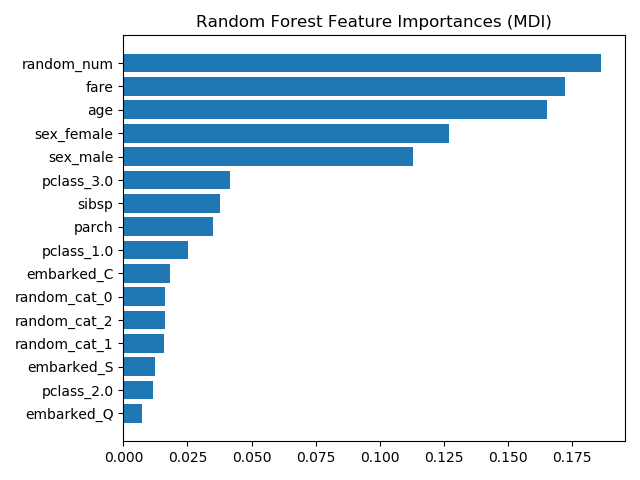# sklearn.compose.ColumnTransformer¶

class sklearn.compose.ColumnTransformer(transformers, *, remainder='drop', sparse_threshold=0.3, n_jobs=None, transformer_weights=None, verbose=False)

[源码]

New in version 0.20.

transformers list of tuples

name str

transformer {‘drop’, ‘passthrough’} or estimator

columns str, array-like of str, int, array-like of int, array-like of bool, slice or callable

remainder {‘drop’, ‘passthrough’} or estimator, default=’drop’

sparse_threshold float, default=0.3

n_jobs int, default=None

transformer_weights dict, default=None

verbose bool, default=False

transformers_ list

named_transformers_Bunch

sparse_output_ bool

>>> import numpy as np>>> from sklearn.compose import ColumnTransformer>>> from sklearn.preprocessing import Normalizer>>> ct = ColumnTransformer(...     [("norm1", Normalizer(norm='l1'), [0, 1]),...      ("norm2", Normalizer(norm='l1'), slice(2, 4))])>>> X = np.array([[0., 1., 2., 2.],...               [1., 1., 0., 1.]])>>> # Normalizer scales each row of X to unit norm. A separate scaling>>> # is applied for the two first and two last elements of each>>> # row independently.>>> ct.fit_transform(X)array([[0. , 1. , 0.5, 0.5],       [0.5, 0.5, 0. , 1. ]])

fit(self, X[, y]) 使用X拟合所有转换器
fit_transform(self, X[, y]) 拟合所有转换器，转换数据并连接结果
get_feature_names(self) 从所有转换器获取特征名称
get_params(self[, deep]) 获取此估计器的参数
set_params(self, **kwargs) 设置此估计器的参数
transform(self, X) 由每个转换器分别变换X，连接结果
__init__(self, transformers, *, remainder='drop', sparse_threshold=0.3, n_jobs=None, transformer_weights=None, verbose=False)

[源码]

fit(self, X, y=None)

[源码]

X {array-like, dataframe} of shape (n_samples, n_features)

y array-like of shape (n_samples,…), default=None

self ColumnTransformer

fit_transform(self, X, y=None)

[源码]

X {array-like, dataframe} of shape (n_samples, n_features)

y array-like of shape (n_samples,), default=None

X_t {array-like, sparse matrix} of shape (n_samples, sum_n_components)

get_feature_names(self)

[源码]

feature_names list of strings

get_params(self, deep=True)

[源码]

deep bool, default=True

params mapping of string to any

property named_transformers_

set_params(self, **kwargs)

[源码]

self -
transform(self, X)

[源码]

X {array-like, dataframe} of shape (n_samples, n_features)

X_t {array-like, sparse matrix} of shape (n_samples, sum_n_components)

## sklearn.compose.ColumnTransformer使用示例¶